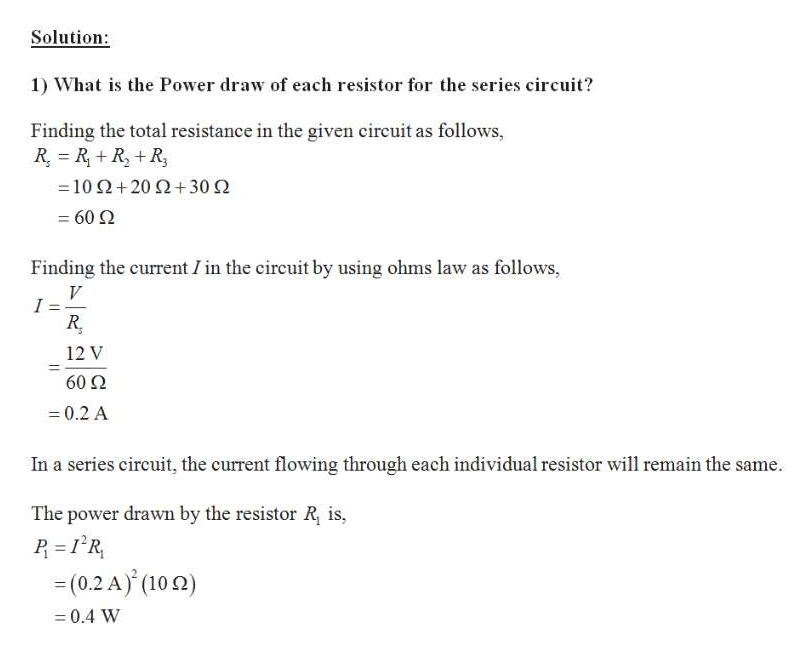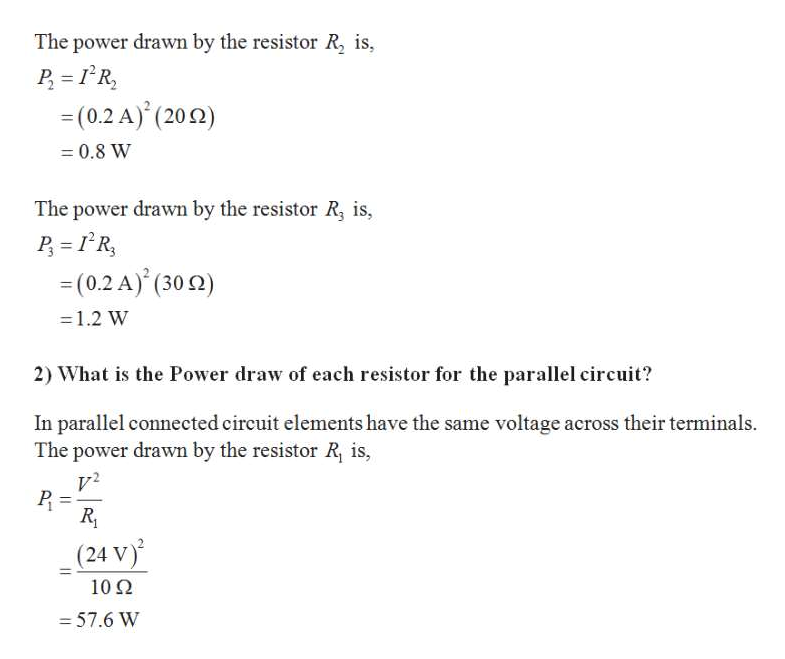# R1 100Vs 12vR2 200Rs =300во

Question
5 views

1.What is the Power draw of each resistor for the series circuit?

2.What is the Power draw of each resistor for the parallel circuit?

check_circle

Step 1help_outlineImage TranscriptioncloseSolution: 1) What is the Power draw of each resistor for the series circuit? Finding the total resistance in the given circuit as follows, R RR+R -10Ω+20 Ω+30 Ω 60 Q Finding the current I in the circuit by using ohms law as follows, I : 12 V 60 Ω = 0.2 A In a series circuit, the current flowing through each individual resistor will remain the same The power drawn by the resistor R, is, (0.2 A) (10) =0.4 W fullscreen
Step 2help_outlineImage TranscriptioncloseThe power drawn by the resistor R, is, P R = (0.2 A) (200) 0.8 W The power drawn by the resistor R, is, R =(0.2 A) (30) 1.2 W 2) What is the Power draw of each resistor for the parallel circuit? In parallel connected circuit elements have the same voltage across their terminals The power drawn by the resistor R, is, (24 V) 102 - 57.6 W fullscreen

### Want to see the full answer?

See Solution

#### Want to see this answer and more?

Solutions are written by subject experts who are available 24/7. Questions are typically answered within 1 hour.*

See Solution
*Response times may vary by subject and question.
Tagged in

### Electrical Engineering